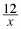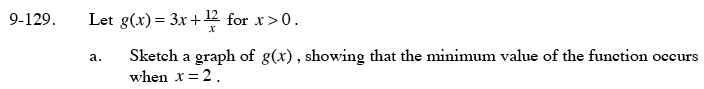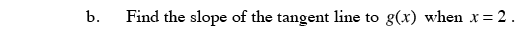### Home > PC > Chapter 9 > Lesson 9.3.3 > Problem9-129

9-129.
1. Let g(x) = 3x +for x > 0. Homework Help ✎

1. Sketch a graph of g(x), showing that the minimum value of the function occurs when x = 2.

2. Find the slope of the tangent line to g(x) when x = 2.